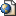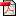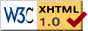Multivariate Gamma based distributions for image processing applications
Dissertation defended on October 25th, 2007, at ENSEEIHT Toulouse (France) in candidacy for the degree of Doctor of Philosophy of the National Polytechnic Institute of Toulouse.

# JuryGérard Letac (LSP - France).Patrice Abry (ENS Lyon - France).Philippe Réfrégier (ECM - France).Cédric Richard (UTT - France).André Ferrari (LUAN - France).Jordi Inglada (CNES - France).Sophie Lambert-Lacroix (LJK - France).Jean-Yves TOURNERET (IRIT/ENSEEIHT/TéSA - France).Jean-Yves TOURNERET (IRIT/ENSEEIHT/TéSA - France).

# Abstract

The wavefront amplitude of many optical systems can be modeled as a sum of incoherent complex components distributed according to Gaussian distributions. The resulting intensity measurements are the sum of the squared modulus of these complex Gaussian components. Parameter estimation and detection problems require to determine accurately the statistical properties of the collected intensities.

The subject of this thesis consists of studying families of multivariate gamma based distributions useful to solve estimation and detection problems in different image processing applications. Multivariate extensions of the uni-dimensional gamma distribution make possible to model the spatial correlations of the observed data as well as the correlation between different images. Therefore, these distributions are potentially interesting for parameter estimation and detection in many applications.

The different kinds of images studied in this thesis are summarized below:

• In synthetic aperture radar (SAR) imaging, the marginal distribution of intensities collected over areas without texture are known to be univariate gamma distributions. Thus, it is natural to introduce multivariate gamma distributions to model the spatial correlations or the dependence between different images. Estimating the correlation coefficient between several images can then be used for image registration or change detection.

• In polarimetric imaging, several measures which correspond to different wavefront polarizations are available. The distributions of the collected intensities are multivariate gamma distribution in the high-flux case and negative multinomial distributions in the low-flux case. Estimating the parameters of these distributions allows us to determine the polarimetric properties of the observed scene.

• In astrophysics, an increasing interest has been shown for the extrasolar planets detection using direct imaging. Methods based on the statistics of a given point of the focal plane have been derived. However these estimation and detection strategies are sub-optimal since the spatial correlation of the wavefront is not taken into account. Multivariate distributions constructed as the diagonal of non-central complex Wishart distributions can be used efficiently to address estimation and detection problems in astrophysic images.

• Furthermore, in the case of low-flux data, i.e. when the exposure times are very short and/or when the intensity of the studied objects are low, the wavefront intensity can not be measured directly. In fact the low values of the intensities lead to consider the photocounting effect. The measures correspond in this case to the number of photons collected on each point of the image. Conditioned upon the corresponding intensity, this number of photons is distributed according to a Poisson distribution with mean proportional to this intensity. The distribution of each photonized data is then a mixed Poisson distribution. Since the intensity vector is distributed according to a multivariate distribution in order to model the intensity correlations, this lead to study multivariate mixed Poisson distributions.

# Award

2008 Léopold Escande Prize.

# MaterialsPdf version of the manuscript (in french).Pdf slides of the defense (in french).

 send me an e-mail Last update : 02/05/2008